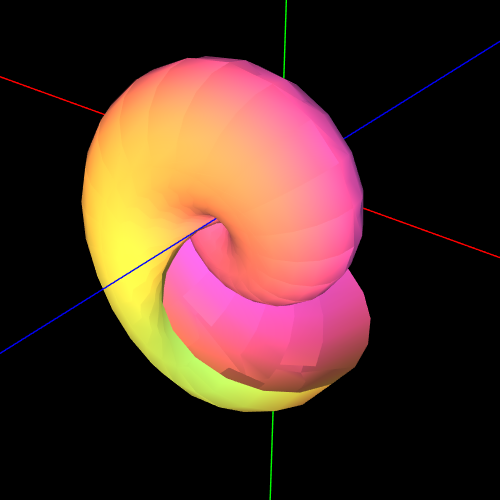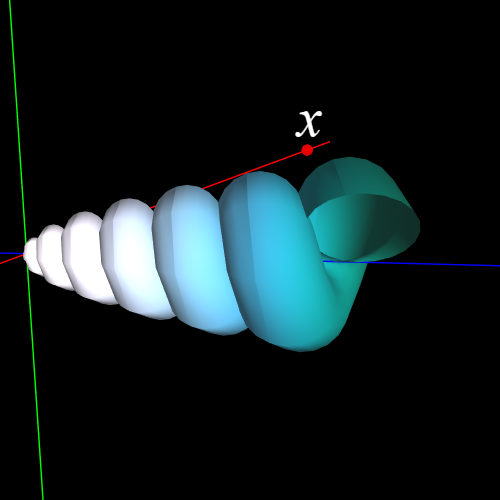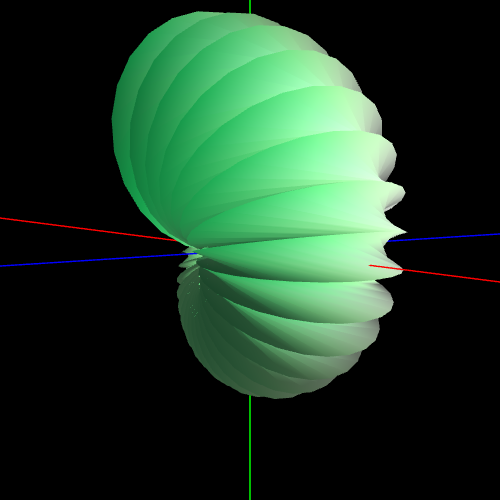#### Drawing a Seashell in iFlow

By Charles Xie

A parametric surface is a 3D surface defined by a set of parametric equations with two parameters, usually denoted as u and v. iFlow can be used to draw any parametric surface. In this article, we show you how to draw a seashell like the following images using this tool.A seashell surface is a surface made by a circle which spirals up the z-axis while decreasing its own radius and distance from the z-axis. It can be defined parametrically as:

x = [u/(2πR)]cos(Nu)[1+cos(v)]
y = [u/(2πR)]sin(Nu)[1+cos(v)]
z = [u/(2πR)]sin(v)+H[u/(2π)]P

where R is the radius, N is the number of turns, H is the height, and P is the power. You can adjust these parameters to make different types of seashells such as a nautilus.

With iFlow, you can use a Parametric Equation block in conjunction with a Surface3D block (do not confuse this with a Space3D block) to create visual code that can generate the seashell surface. You can also use global variables to allow users to adjust the control parameters such that the shape can be varied. An implementation is shown in the image below.